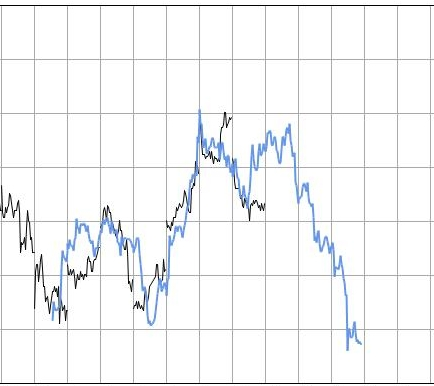# Fractal Projection

The Fractal Projection algorithm is a unique approach to forecasting. It answers all the cases where there is not enough historical data to be able to compute a meaningful forecast and/or where the structure of the historical data is fractal.

## Fractal Data Structure

What do we mean by Fractal Data Structure? Essentially we are referring to:

• Data has a semi-periodic structure.
• If we analyze separately each period of data its structure is similar to the bigger/parent period.

One of the classical examples of Fractal Data is the Stock Market where you can find evidence of both a periodic structure and of a recursive substructure.

## Projection

The projection algorithm takes as input the pattern to be projected and then stretches either horizontally or vertically the pattern interpolating as needed the data to produce a meaningful output. Much of the hard data mining work is devoted to finding the right pattern in the raw data to project forward but once that one is found or computed then it can be projected forward on any timeframe.

## Example

This example is drawn from an intraday stock chart:The picture clarifies how the projection can work exploiting the recursive nature of the Stock Market. Of course the same algorithm can be applied to Sales Forecasting or wherever the two premises are applicable.

 Forecasting Methods Holt Winter’s, Series Decomposition and Wavelet Benchmarks Time Series Forecasting Use of the Moving Average in Time-series Forecasting Forecasting Concepts Denoising Techniques Error Statistics Computational Performance Fast Fourier Transform Moving Averages Kernel Smoothing Active Moving Average Savitsky-Golay Smoothing Fractal Projection Downloading Financial Data from Yahoo Multiple Regression Digital Signal Processing Principal Component Analysis Curve Analysis Options Pricing with Black-Scholes Markowitz Optimal Portfolio Time-series preprocessing